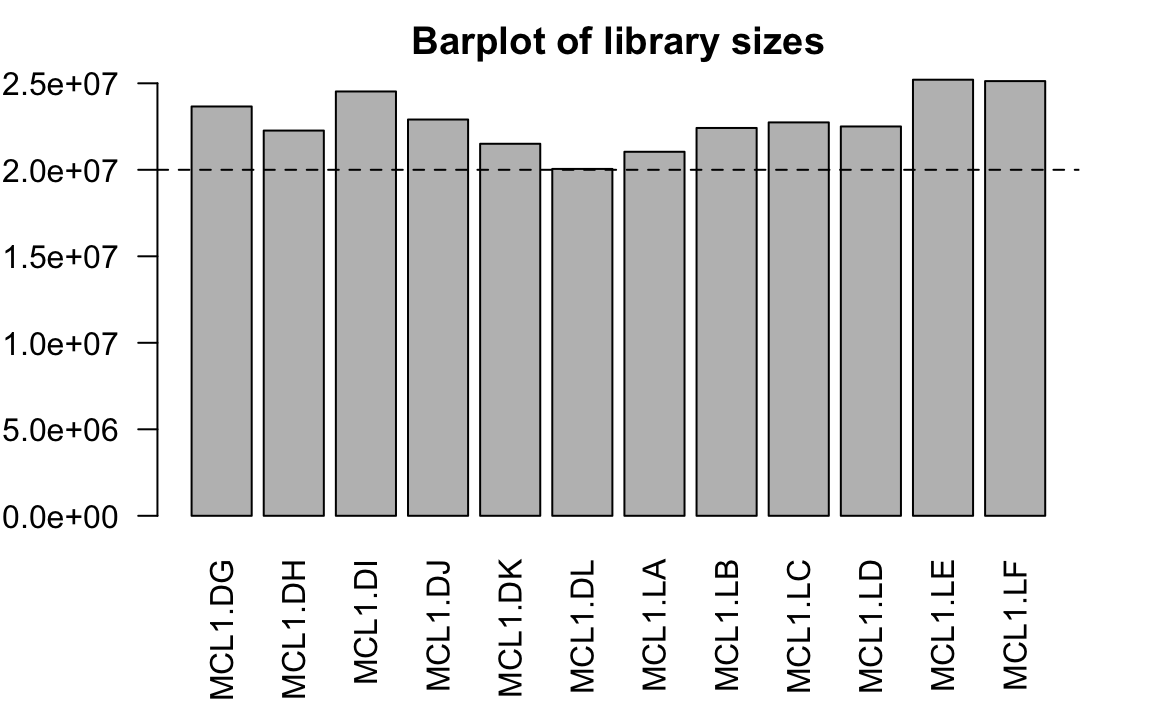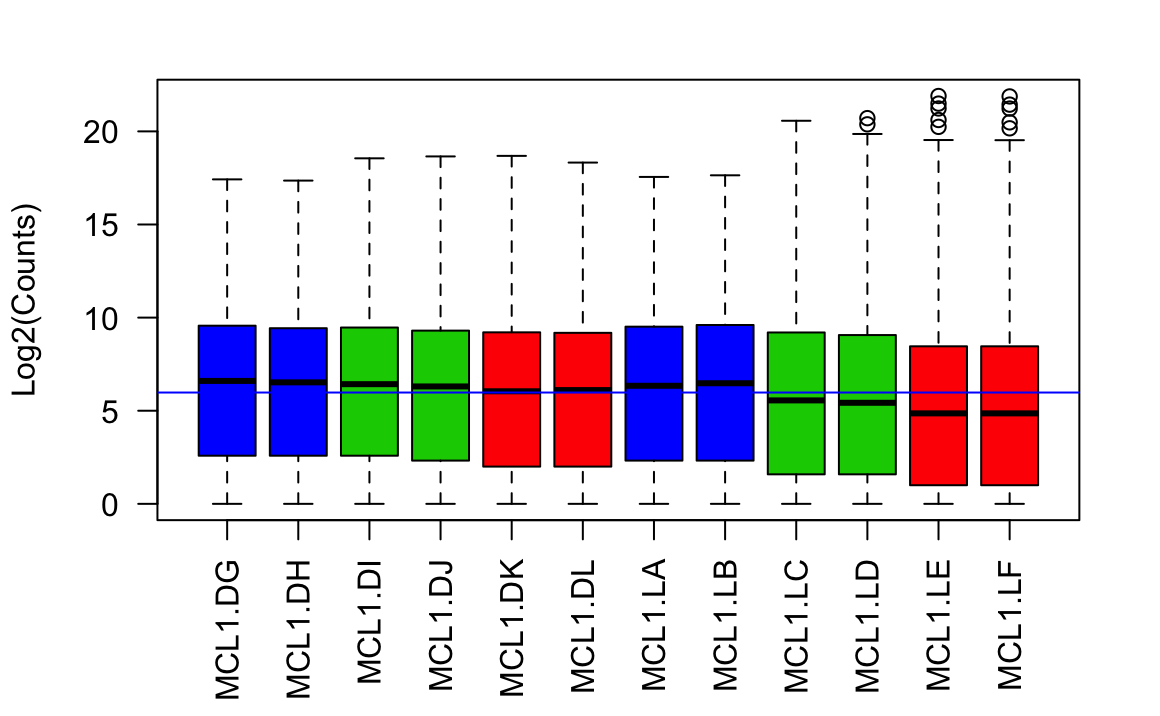# Introduction

In this section we will begin the process of analysing the RNAseq in R. In the next section we will use DESeq2 for differential analysis. Before we do that we need to:

• import our counts into R
• manipulate the imported data so that it is in the correct format for DESeq2
• filter out unwanted genes
• run some initial QC on the raw count data

We will also look at the effects of normalisation for composition bias.

A detailed analysis workflow, recommended by the authors of DESeq2 can be found on the Bionconductor website.

# Data import

First, let’s load all the packages we will need to analyse the data.

``````library(DESeq2)
library(tidyverse)``````
``package ‘dplyr’ was built under R version 3.5.1``

## Mouse mammary gland dataset

The data for this tutorial comes from a Nature Cell Biology paper, EGF-mediated induction of Mcl-1 at the switch to lactation is essential for alveolar cell survival (Fu et al. 2015). The raw data (sequence reads) can be downloaded from SRA under SRP045534, and processed data (counts) can be downloaded from Gene Expression Omnibus database (GEO) under accession number GSE60450. Please see Supplementary material for instructions on downloading raw files from SRA and aligning fastq using HISAT2.

This study examines the expression profiles of basal stem-cell enriched cells (B) and committed luminal cells (L) in the mammary gland of virgin, pregnant and lactating mice. Six groups are present, with one for each combination of cell type and mouse status. Each group contains two biological replicates.

The `sampleinfo` file contains basic information about the samples that we will need for the analysis today.

``````# Read the sample information into a data frame
sampleinfo``````

## Reading in the count data

The raw reads were aligned using HISAT2 (Kim, Langmead, and Salzberg 2015) to the GRCm38 mouse reference genome from Ensembl. featureCounts (Liao, Smyth, and Shi 2014) was used to count reads against the Ensembl gene annotation and generate a counts matrix (as described in Section 1).

First we need to read the data into R from the file in the data directory.

``````# Read the data into R
comment = "#",
stringsAsFactors=F)

In the `seqdata` object each row represents a gene. The columns contains:

1 Geneid - Ensembl ID
2-5 Chr, Start, End, Strand - Genomic locations of exons of the gene
6 Length - Transcript length of the gene
7-18 One column for each sample with the count of how many reads were assigned
to each gene by featureCounts.

### A quick intro to `dplyr`

One of the most complex aspects of learning to work with data in `R` is getting to grips with subsetting and manipulating data tables. The package `dplyr` (Wickham et al. 2018) was developed to make this process more intuitive than it is using standard base `R` processes. It also makes use of a new symbol `%>%`, called the “pipe”, which makes the code a bit tidier.

We are introducing this because it makes many of the processes we will look at later much simpler. Importantly it also results in code that is much easier to read and understand.

Let’s have quick look at this by playing with our `sampleinfo` table.

Suppose we wanted a new sample table that:

1. Just includes the “basal” samples
2. Only has the columns “CellType” and “Group”
3. Renames the “CellType” column as “Cell”

In base `R` we would do the something like:

``````newTable <- sampleinfo

basal <- which(newTable\$CellType=="basal")
newTable <- newTable[basal, ]

newTable <- newTable[basal, c("CellType", "Group")]

colnames(newTable) <- "Cell"``````

With `dplyr` we can use two new functions - `filter` and `select` - for the first two steps:

``````newTable <- sampleinfo
newTable <- filter(newTable, CellType=="basal")
newTable <- select(newTable, CellType, Group)``````

There’s no need to quote the column names as dplyr intelligently interprets the arguments it’s passes as belonging to the data table columns.

Rather than repeatedly reassigning `newTable <- f(newTable)` as above, we can use the pipe - `%>%`. This takes the output of one function and “pipes” it into the first argument of the next function so that we don’t have to keep specifying the object we are working with:

``````newTable <- sampleinfo %>%
filter(CellType=="basal") %>%
select(CellType, Group) %>%
rename(Cell=CellType)``````

This is a fairly trivial example and the benefits may not be immediately obvious, but once you get used to using `dplyr` (and the other related “tidyverse” packages, such as `stringr`) you’ll find it much more powerful and easy to use than base R.

We will encounter a few more `dplyr` commands during the course, we will explain their use as we come to them.

## Format the data

We will be manipulating and reformating the counts matrix into a suitable format for DESeq2.

The `seqdata` is a `dataframe` in which the first six columns contain annotation information and the remaining columns contain the count data.

DESeq2 requires a simple object containing only the count data, we’ll keep the gene ID by setting them as the row names.

Let’s create new counts data object, `countdata`, that contains only the counts for the 12 samples.

Our `sampleinfo` object contains a column with the sample names. We should adjust the column names of our matrix to match them - we just need to remove the `.bam` suffix.

It is also critical to ensure that the samples in the columns are in the same order as the rows of `sampleinfo`. When we load these objects into DESeq2 for the analysis it will not guess which row of the `sampleinfo` belongs to which column of the counts matrix, it will assume the same order.

We’ll use to new `dplyr` commands:

• `columns_to_rownames` to set the rownames using a named column
• `rename_all` which allows to rename all the columns using a string function
``````countdata <- seqdata %>%
column_to_rownames("Geneid") %>% # turn the geneid column into rownames
rename_all(str_remove, ".bam") %>% # remove the ".bam" from the column names
select(sampleinfo\$Sample) %>% # keep sample columns using sampleinfo\$Sample
as.matrix()
``````                   MCL1.DG MCL1.DH MCL1.DI MCL1.DJ MCL1.DK MCL1.DL MCL1.LA
ENSMUSG00000102693       0       0       0       0       0       0       0
ENSMUSG00000064842       0       0       0       0       0       0       0
ENSMUSG00000051951     665     446      86     361     559     447       0
ENSMUSG00000102851       0       0       0       0       0       0       0
ENSMUSG00000103377       0       0       0       0       1       0       0
ENSMUSG00000104017       0       0       0       0       0       0       0
MCL1.LB MCL1.LC MCL1.LD MCL1.LE MCL1.LF
ENSMUSG00000102693       0       0       0       0       0
ENSMUSG00000064842       0       0       0       0       0
ENSMUSG00000051951       0       0       0       2       0
ENSMUSG00000102851       0       0       0       0       0
ENSMUSG00000103377       0       0       0       0       0
ENSMUSG00000104017       0       0       0       0       0``````

Here, we used `str_remove` to remove the unwanted suffix from the column names. The `stringr` package has a lots of useful functions for manipulating strings (text), e.g. `str_replace` or `str_extract`.

# Filtering the genes

For many analysis methods it is advisable to filter out as many genes as possible prior to starting the analysis in order to decrease the impact on fasle discovery rates when applying multiple testing correction. This is normally done by filtering out genes with low numbers of reads, which are likely to be uninformative.

With `DESeq` this is not necessary as it applies a process it calls `independent filtering` during the analysis process. On the other hand, some filtering for genes that are very lowly expressed does reduce the size of the data matrix, meaning that less memory is required and processing steps are carried out faster.

We will keep all genes where the total number of reads across all samples is greater than 5.

``dim(countdata)``
`` 45513    12``
``````keep <- rowSums(countdata) > 5
countdata <- countdata[keep,]
dim(countdata)``````
`` 22013    12``

# Quality assessment

Before moving on to doing the actually differential expression analysis it important do assess the quality of our data.

## Library sizes bar plot

First, we can plot how many reads we have for each sample. Whilst normalisation can account for imbalance in coverage across the samples, extreme differences may be indicative of underlying problems in the samples.

``````librarySizes <- colSums(countdata)
barplot(librarySizes,
names=names(librarySizes),
las=2,
main="Barplot of library sizes")
abline(h=20e6, lty=2)``````## Count distribution boxplots

Count data is not normally distributed, so if we want to examine the distributions of the raw counts it is helpful to transform the data on to a log scale. Typically we use a `log2` transformation, however, because the data is count data and will contain many `0`s we need to add a count of 1 to every value in order to prevent attempting `log2(0)` from creating errors.

``````# Get log2 counts per million
logcounts <- log2(countdata + 1)``````

We’ll check the distribution of read counts using a boxplot and well add some colour to see if there is any difference between sample groups.

``````# make a colour vector
statusCol <- as.numeric(factor(sampleinfo\$Status)) + 1
# Check distributions of samples using boxplots
boxplot(logcounts,
xlab="",
ylab="Log2(Counts)",
las=2,
col=statusCol)
# Let's add a blue horizontal line that corresponds to the median logCPM
abline(h=median(as.matrix(logcounts)), col="blue")``````From the boxplots we see that overall the density distributions of raw log-intensities are not identical but still not very different. If a sample is really far above or below the blue horizontal line we may need to investigate that sample further.

### Challenge 1

1. Use the `DESeq2` function `rlog` to transform the count data. This function also normalises for library size.
2. Plot the count distribution boxplots with this data How has this effected the count distributions?

## Principle Component Analysis

A principle components analysis (PCA) is an example of an unsupervised analysis, where we don’t specify the grouping of the samples. If your experiment is well controlled and has worked well, we should that replicate samples cluster closely, whilst the greatest sources of variation in the data should be between treatments/sample groups. It is also an incredibly useful tool for checking for outliers and batch effects.

To run the PCA we should first normalise our data for library size and transform to a log scale.DESeq2 provides two commands that can be used to do this, here we will use the command `rlog`. `rlog` performs a log2 scale transformation in a way that compensates for differences between samples for genes with low read count and also normalizes between samples for library size.

You can read more about `rlog`, it’s alternative `vst` and the comparison between the two here.

To plot the PCA results we will use the `autoplot` function from the `ggfortify` package (Tang, Horikoshi, and Li 2016). `ggfortify` is built on top of `ggplot2` and is able to recognise common statistical objects such as PCA results or linear model results and automatically generate summary plot of the results in an appropriate manner.

``````library(ggfortify)
rlogcounts <- rlog(countdata)
# run PCA
pcDat <- prcomp(t(rlogcounts))
# plot PCA
autoplot(pcDat)``````